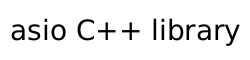Timer.4 - Using a member function as a handler

In this tutorial we will see how to use a class member function as a callback handler. The program should execute identically to the tutorial program from tutorial Timer.3.

#include <iostream>
#include <asio.hpp>
#include <boost/bind/bind.hpp>

Instead of defining a free function print as the callback handler, as we did in the earlier tutorial programs, we now define a class called printer.

class printer
{
public:

The constructor of this class will take a reference to the io_context object and use it when initialising the timer_ member. The counter used to shut down the program is now also a member of the class.

printer(asio::io_context& io)
: timer_(io, asio::chrono::seconds(1)),
count_(0)
{

The boost::bind function works just as well with class member functions as with free functions. Since all non-static class member functions have an implicit this parameter, we need to bind this to the function. As in tutorial Timer.3, boost::bind converts our callback handler (now a member function) into a function object that can be invoked as though it has the signature void(const asio::error_code&).

You will note that the asio::placeholders::error placeholder is not specified here, as the print member function does not accept an error object as a parameter.

timer_.async_wait(boost::bind(&printer::print, this));
}

In the class destructor we will print out the final value of the counter.

~printer()
{
std::cout << "Final count is " << count_ << std::endl;
}

The print member function is very similar to the print function from tutorial Timer.3, except that it now operates on the class data members instead of having the timer and counter passed in as parameters.

void print()
{
if (count_ < 5)
{
std::cout << count_ << std::endl;
++count_;

timer_.expires_at(timer_.expiry() + asio::chrono::seconds(1));
timer_.async_wait(boost::bind(&printer::print, this));
}
}

private:
int count_;
};

The main function is much simpler than before, as it now declares a local printer object before running the io_context as normal.

int main()
{
asio::io_context io;
printer p(io);
io.run();

return 0;
}

See the full source listing